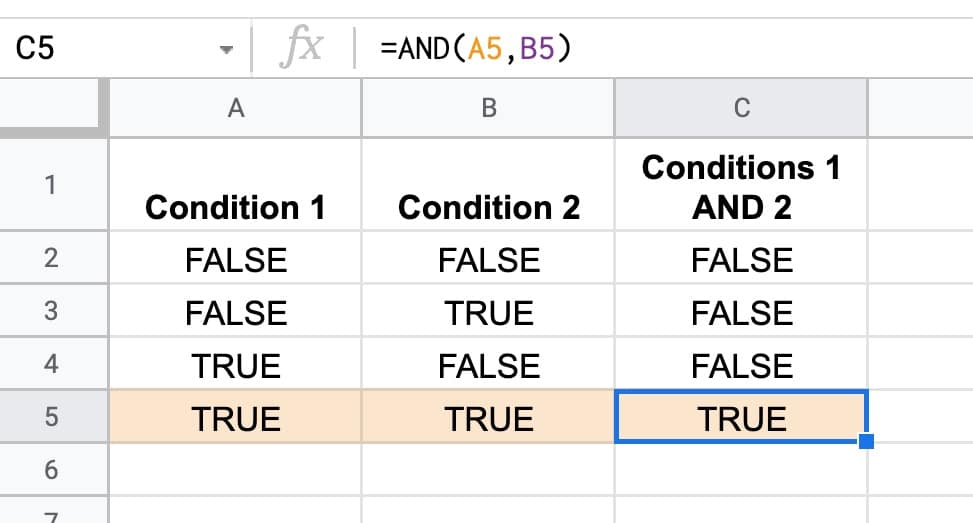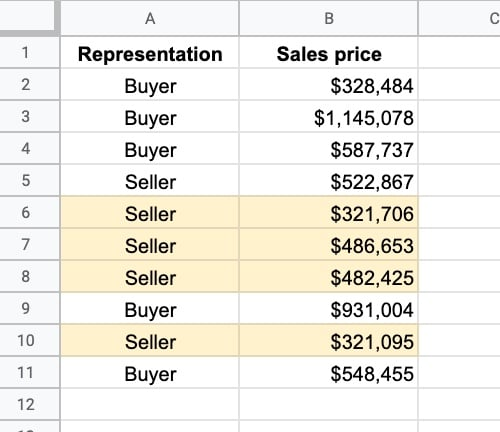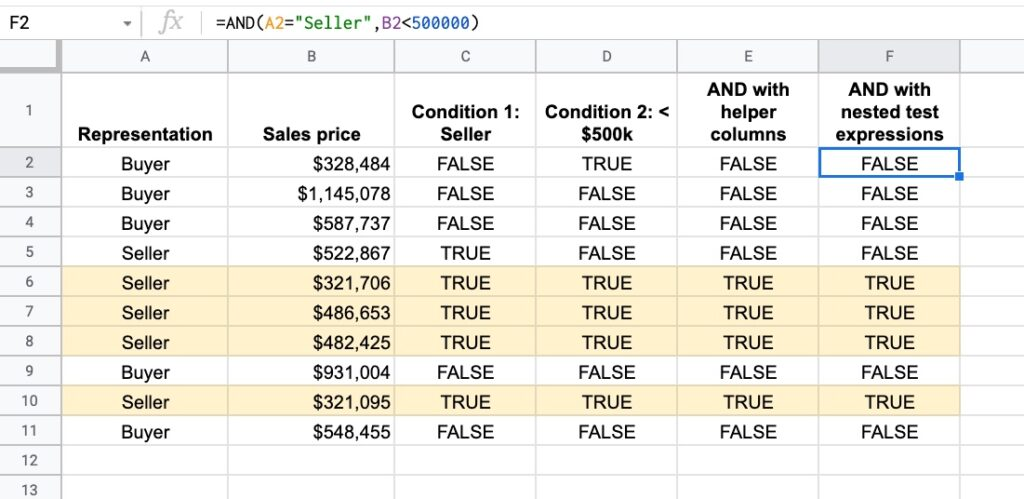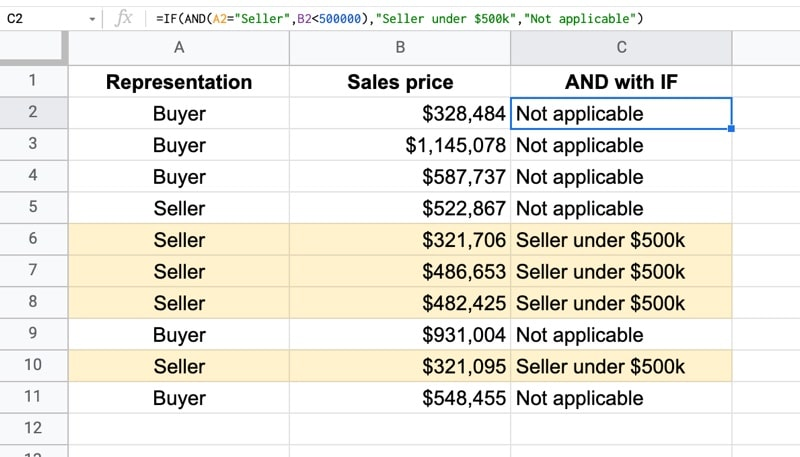# The AND Function in Google Sheets

The AND function in Google Sheets is a logical function that outputs TRUE if all of the inputs are TRUE, and FALSE otherwise.It has useful applications in data cleaning where it pairs well with the IF Function and OR Function to categorize and organize data.

## AND Formula Example

Suppose we have the following dataset:We want to find all the rows that have “Seller” in column A and values less than \$500,000 in column B. I’ve highlighted them yellow for illustration.

So the first test expression, in cell C2, is:

`=A2 = "Seller"`

And the second test expression, in cell D2, is:

`=B2 < 500000`

The results of these two test expressions can then be fed into an AND formula:

`=AND(C2:D2)`

or

`=AND(C2,D2)`

Both of these AND formulas produce the same result. You can enter ranges or cells of data.

We can also drop the two expressions directly into AND, like this:

`=AND(A2 = "Seller" , B2 < 500000)`

All of these AND formulas will output TRUE only when both of the inputs are TRUE, otherwise, the output will be FALSE.The yellow rows all have “Seller” in column A and a value less than \$500k in column B.

## AND Function Syntax

`=AND(logical_expression1, [logical_expression2, ...])`

It takes one or more arguments, which can be cells, ranges, or expressions containing logical tests.

It’s part of the Logical family of functions in Google Sheets, along with the OR function.

### Function Notes

Let’s look at the AND function inputs more closely:

Expression Inputs

Expression inputs are tests that result in TRUE or FALSE values.

For example, here are some expressions that output a TRUE or FALSE result and can therefore be inserted into an AND formula.

`A1 = "Some Value"`
`B1 > 100`
`ISEMAIL(A1)`
`A1 < B1`
`ISEVEN(A1)`

These expressions can be in their own cells and referenced in the AND formula like this:

`=AND(C1,D1)`

or they can be dropped directly into AND like this:

`=AND(A1 = "Some Value",B1 > 100)`

Number Inputs

0 is interpreted as FALSE by the AND Function. All other numbers are interpreted as TRUE.

So `AND(1,1)` is TRUE. But `AND(1,0)` is FALSE.

Text Inputs

Generally ignored by the AND function although there is some nuance around this. See the Twitter thread at the bottom of this post.

## AND Function Template

Feel free to make a copy: File > Make a copy...

If you can't access the template, it might be because of your organization's Google Workspace settings. If you right-click the link and open it in an Incognito window you'll be able to see it.

## AND + IF Function

AND pairs nicely with the IF Function because the TRUE/FALSE output from the AND formula feeds directly into the first argument of the IF function.

Using the same example as earlier, suppose we want to label the Sellers under \$500k in our dataset.

We simply put the AND formula from earlier into the first argument of our IF function:

`=IF(AND(A2 = "Seller" , B2 < 500000) , "Seller under \$500k" , "Not applicable" )`

The output of this formula is: